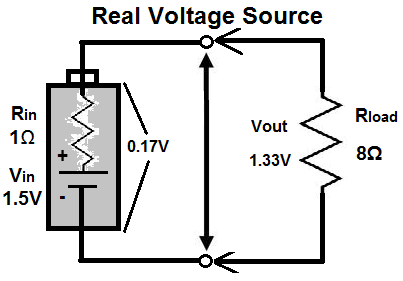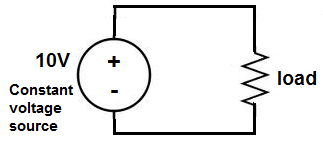﻿ What is a Constant Voltage Source? ﻿# What is a Constant Voltage Source?A constant voltage source is a power source which provides a constant voltage to a load, even despite changes and variance in load resistance.

In other words, the voltage which a constant voltage source provides is steady, even if the resistance of the load varies.

A constant voltage source is, thus, a very valuable component because it can supply steady voltage even if there are changes in resistance, even a wide variance in the resistance. This comes in use when a circuit needs a steady voltage supply, without fluctuations.

The graph below represents the voltage which comes from a constant voltage source.You can see that the voltage is constant all throughout despite changes in current or resistance.

### How Does A Constant Voltage Source Work?

A constant voltage source is a power generator whose internal resistance is very low compared with the load resistance it is giving power to. Because its internal resistance is so low, it dumps most of its voltage across the higher resistance load. Remember that according to ohm's law, voltage is equal to current x resistance (V=IR). So voltage is dropped across the higher resistance component. If the resistance of the voltage source is practically zero, then instead of dropping its voltage across itself, it will drop it across the load entirely instead.

Thus, a constant voltage source follows the rules of voltage division. Being that it has very low internal resistance and the load resistance is much higher, the voltage will practically drop entirely across the load.

Look at the following voltage divider circuit below:Notice how this voltage source, shown above, supplies 1.5V, in total, from out of it. The majority of this 1.5 volts drops across the resistor of greater resistance, which is 8Ω; 1.33V of the 1.5V drops across the load. The remaining 0.17V drops across the battery which has a resistance of 1Ω.

Now let's decrease the resistance of the voltage source so that now it has a resistance of 0Ω. The below voltage source represents a voltage source which has zero internal resistance.Because the resistance is 0Ω and the load is 8Ω, all of the voltage drops across the 8Ω load resistor. Greater voltage will always drop across the component with the higher resistance.

This is how constant voltage sources work.

### Constant Voltage Source Circuit

A constant voltage source circuit is just a constant voltage source connected to the load which it powers.This load above will have a constant voltage of 10V supplied to it regardless of whether the load resistance varies.

Related Resources

HTML Comment Box is loading comments...